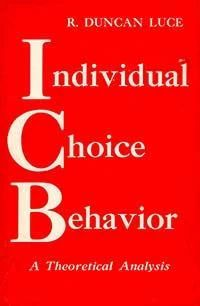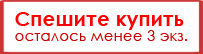# Individual Choice Behavior. A Theoretical Analysis (букинист)500 ₽
Выберите носитель:

Печ. книга

Аудио-книга
Формат книги: крупный, 15.2 на 23.3 см

Издательство: John Wiley & Sons

Страниц: 154

Код: 23890

#### Аннотация:

This research monograph is devoted to a theoretical (mathematical) analysis of one of the major themes of interest to psychologists: choice. The analysis begins by stating a general axiom that may hold among the probabilities of choice from related sets of alternatives. This is shown to imply the existence of a ratio scale that is then used to analyze a number of traditional problems. The first subject treated is psychophysics and covers areas involving:

• time-and space-order effects

• Fechner’s equal jnd problem

• power law in psychophysics and its relation to discrimination data

• psychophysical interaction between two independent physical variables and possible correlates with Stevens’ distinction between prothetic and metathetic continua

• Thurston’s law of comparative judgment

• signal detectability theory

• ranking of stimuli

The next major theme studied is utility theory. Unusual results are obtained which suggest an experiment to test the theory. Topics in learning are analyzed in a concluding chapter which uses the stochastic theories of learning as the basic approach with the exception that distributions of response strengths are assumed to be transformed rather than response probabilities. The author arrives at three classes of learning operators, both linear and non-linear.